CAT  >  Maths and Logical Reasoning Test- 6

# Maths and Logical Reasoning Test- 6

Test Description

## 15 Questions MCQ Test TISS-NET Section Wise & Full Mock Test Series | Maths and Logical Reasoning Test- 6

Maths and Logical Reasoning Test- 6 for CAT 2022 is part of TISS-NET Section Wise & Full Mock Test Series preparation. The Maths and Logical Reasoning Test- 6 questions and answers have been prepared according to the CAT exam syllabus.The Maths and Logical Reasoning Test- 6 MCQs are made for CAT 2022 Exam. Find important definitions, questions, notes, meanings, examples, exercises, MCQs and online tests for Maths and Logical Reasoning Test- 6 below.
Solutions of Maths and Logical Reasoning Test- 6 questions in English are available as part of our TISS-NET Section Wise & Full Mock Test Series for CAT & Maths and Logical Reasoning Test- 6 solutions in Hindi for TISS-NET Section Wise & Full Mock Test Series course. Download more important topics, notes, lectures and mock test series for CAT Exam by signing up for free. Attempt Maths and Logical Reasoning Test- 6 | 15 questions in 15 minutes | Mock test for CAT preparation | Free important questions MCQ to study TISS-NET Section Wise & Full Mock Test Series for CAT Exam | Download free PDF with solutions
 1 Crore+ students have signed up on EduRev. Have you?
Maths and Logical Reasoning Test- 6 - Question 1

### Evaluate the following: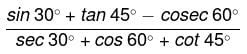Detailed Solution for Maths and Logical Reasoning Test- 6 - Question 1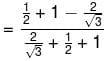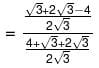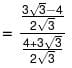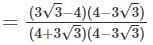[Dividing and multiplying by (4−3√3) ]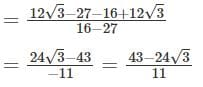Hence, the correct option is (B).

Maths and Logical Reasoning Test- 6 - Question 2

### In △ABC,O is the incentre and ∠BOC= 135°. The measure of ∠BAC is:

Detailed Solution for Maths and Logical Reasoning Test- 6 - Question 2

In △ABC,O is the incentre and ∠BOC= 135°.

Incentre of a triangle: The point of intersection of all three angle bisectors of a triangle is called its in - centre.

The distance between In - centre and its all three sides is always equal and its called inradius.

∠BOC is the incentre of △ABC.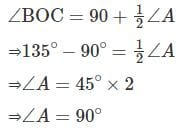Hence, the correct option is (C).

Maths and Logical Reasoning Test- 6 - Question 3

### A man sells an article on Rs. 280 and gets 40% profit. Then find the cost price of the article.

Detailed Solution for Maths and Logical Reasoning Test- 6 - Question 3

Given:

The selling price of the article = Rs. 280

The profit obtained = 40%

Selling price = cost price ×(100+ profit) %

Let the cost price be CP.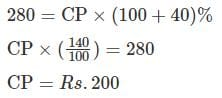∴ The cost price of the article is Rs. 200.

Hence, the correct option is (B).

Maths and Logical Reasoning Test- 6 - Question 4

The product of two numbers is x/y If one of the numbers is x/y2 then the other number is:

Detailed Solution for Maths and Logical Reasoning Test- 6 - Question 4

Given:

The product of two numbers is y/x.

One of the number is x/y2

Let the other number be M.

Then,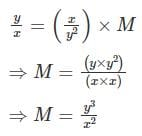Therefore, the other number is y3/x2.

Hence, the correct option is (A).

Maths and Logical Reasoning Test- 6 - Question 5

A car travels a certain distance at 70 km/h and comes back at 30 km/h. Find the average speed for total journey.

Detailed Solution for Maths and Logical Reasoning Test- 6 - Question 5

Given:

The Car goes with the speed (S1) = 70 km/hr

The car comes back with the speed (S2) = 30 km/hr

We know that, Average speed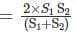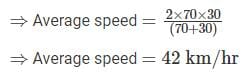Hence, the correct option is (A).

Maths and Logical Reasoning Test- 6 - Question 6

At what rate of interest will a sum of Rs. 4,500 amount to Rs. 6,525 at simple interest for 5 years?

Detailed Solution for Maths and Logical Reasoning Test- 6 - Question 6

Given-

Principal = Rs. 4500

Amount = Rs. 6525

We know that, Interest =Amount - Principal

⇒ Interest =6525−4500

=2025

According to Condition,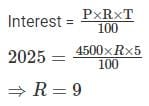∴ The rate of interest is 9%.

Hence, the correct option is (B).

Maths and Logical Reasoning Test- 6 - Question 7

Choose the odd numerical pair/group in the following options.

Detailed Solution for Maths and Logical Reasoning Test- 6 - Question 7

All except 55 - 62 have difference of nine between them.

(A) 55 – 62 = difference is 7

(B) 34 – 43 = difference is 9

(C) 62 –71 = difference is 9

(C) 83 – 92 = difference is 9

Hence, the correct option is (A).

Maths and Logical Reasoning Test- 6 - Question 8

In the given matrix which option will replace the question mark.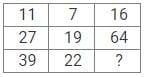Detailed Solution for Maths and Logical Reasoning Test- 6 - Question 8

The logic follows here is :-

From the first row :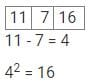From second row :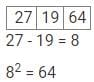From Third row :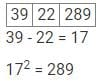So, that Third column third row is "289".

Hence, the correct option is (C).

Maths and Logical Reasoning Test- 6 - Question 9

Four letter clusters have been given, out of which three are alike in some manner, while one is different. Select the odd letter cluster.

Detailed Solution for Maths and Logical Reasoning Test- 6 - Question 9

The pattern followed here is: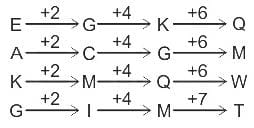All follow the same pattern except “GIMT”.

Hence, the correct option is (C).

Maths and Logical Reasoning Test- 6 - Question 10

Evaluate: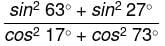Detailed Solution for Maths and Logical Reasoning Test- 6 - Question 10

Given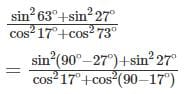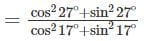[∵ sin (900 - ?) = cos ? and cos (900 - ?) = sin ? ]

= 1/1 = 1 [∵ sin2? + cos2? = 1]

Maths and Logical Reasoning Test- 6 - Question 11

Two numbers are respectively 20% and 50% of a third number. What percent is the first number of the second?

Detailed Solution for Maths and Logical Reasoning Test- 6 - Question 11

Given:

Two numbers are respectively 20% and 50% of a third number.

Let the third number be x.

According to the question; First number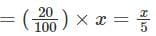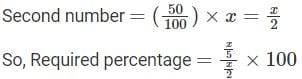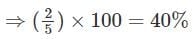∴ The correct answer is 40%.

Hence, the correct option is (D).

Maths and Logical Reasoning Test- 6 - Question 12

11 \$ 11 = 99, 45 \$ 15 = 615, 44 \$ 12 = 472, then find the value of 32 \$ 11 = ?

Detailed Solution for Maths and Logical Reasoning Test- 6 - Question 12

The pattern/logic followed here is:

11 \$ 11 = 99 → (11 × 11) - (11 + 11) = 121 - 22 = 99;

45 \$ 15 = 615 → (45 × 15) - (45 + 15) = 675 - 60 = 615;

44 \$ 12 = 472 → (44 × 12) - (44 + 12) = 528 - 56 = 472;

Similarly,

32 \$ 11 = ? → (32 × 11) - (32 + 11) = 352 - 43 = 309

Hence, the correct option is (D).

Maths and Logical Reasoning Test- 6 - Question 13

In triangle ABC, right-angled at B, if tan A = 1/√3, find the value of:

SinA cos C + cos A sin C

Detailed Solution for Maths and Logical Reasoning Test- 6 - Question 13

Let us draw a triangle ABC,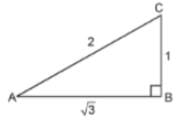tan A = 1/√3

Where, BC=1 and AB = √3

In △ABC,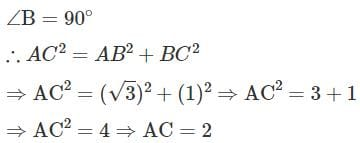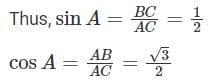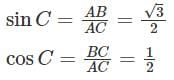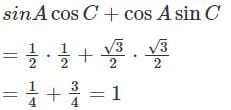Hence, the correct option is (A).

Maths and Logical Reasoning Test- 6 - Question 14

The LCM of two numbers is 90 times their HCF. The sum of LCM and HCF is 1456 . If one of the number is 160 , then what is the other number?

Detailed Solution for Maths and Logical Reasoning Test- 6 - Question 14

Let the HCF of two numbers be x.

∴ LCM of two numbers be 90x.

According to the question,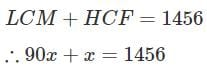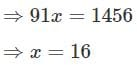∴ HCF of two numbers =16

and LCM of two numbers =90×16

= 1440

We know that, LCM ×HCF= Product of two numbers

⇒ 1440 x 16 = 160 x second number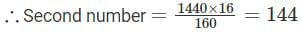Hence, the correct option is (C).

Maths and Logical Reasoning Test- 6 - Question 15

In the following question, select the related letters from the given alternatives.

OPEN : PRHR : : CLOSE : ?

Detailed Solution for Maths and Logical Reasoning Test- 6 - Question 15

The pattern followed here is: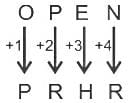So,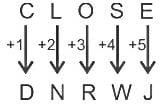Hence, the correct option is (A).

## TISS-NET Section Wise & Full Mock Test Series

28 tests
 Use Code STAYHOME200 and get INR 200 additional OFF Use Coupon Code
Information about Maths and Logical Reasoning Test- 6 Page
In this test you can find the Exam questions for Maths and Logical Reasoning Test- 6 solved & explained in the simplest way possible. Besides giving Questions and answers for Maths and Logical Reasoning Test- 6, EduRev gives you an ample number of Online tests for practice

## TISS-NET Section Wise & Full Mock Test Series

28 tests

### How to Prepare for CAT

Read our guide to prepare for CAT which is created by Toppers & the best Teachers